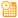# Parse Date Time

 Block Group: Date Time Operations Icon:The Parse Date Time block converts a serial number, or a date and time string, to multiple outputs that represent the serial number, year, month, day, hour, minute, second, millisecond, and weekday.

For information on using dataflow blocks, see Dataflow.

## Input/Output Property

The following property of the Parse Date Time block can take input and give output.

• input (number or string)

input specifies the serial number or any supported date time string as outlined here. This can also be text from which a date can be parsed.

## Output Properties

The following properties of the Parse Date Time block can give output but cannot take input.

• time (number)
• year (number)
• month (number)
• day (number)
• hour (number)
• minute (number)
• second (number)
• millisecond (number)
• weekday (number)

time returns the serial number.

year returns the year.

month returns the month of the year as a number from 1 to 12.

day returns the day of the month as a number from 1 to 31.

hour returns the hour of the day as a number from 0 to 23.

minute returns the minute of the hour as a number from 0 to 59.

second returns the second of the minute as a number from 0 to 59.

millisecond returns the millisecond of the second as a number from 0 to 999.

weekday returns the day of the week as a number from 1 to 7. The number 1 represents Sunday, and the number 7 represents Saturday.

## Examples

The following image shows three examples of the Parse Date Time block. The leftmost Parse Date Time block extracts a date string from text. The middle Parse Date Time block takes a serial number as input. The rightmost Parse Date Time block takes a date string as input.